# RD Sharma Solutions Class 10 Chapter 12 Some Applications of Trigonometry Exercise 12.1

Here you can get free RD Sharma Solutions for Class 10 Maths Chapter 12 Some Applications of Trigonometry Exercise 12.1. All RD Sharma Book Solutions are given here exercise wise for the chapter Some Applications of Trigonometry. RD Sharma Solutions are helpful in the preparation of several school level, graduate and undergraduate level competitive exams. Practicing questions from RD Sharma Mathematics Solutions for Class 10 Chapter 12 Some Applications of Trigonometry is proven to enhance your math skills.

 Class: Class 10th Chapter: Chapter 12 Exercise: Exercise 12.1 Name: Some Applications of Trigonometry

## RD Sharma Solutions Class 10 Chapter 12 Some Applications of Trigonometry Exercise 12.1

RD Sharma Class 10 Solutions Chapter 12 for Some Applications of Trigonometry Exercise 12.1 are given below.

RD Sharma Solutions Class 10 Chapter 12 Some Applications of Trigonometry Exercise 12.1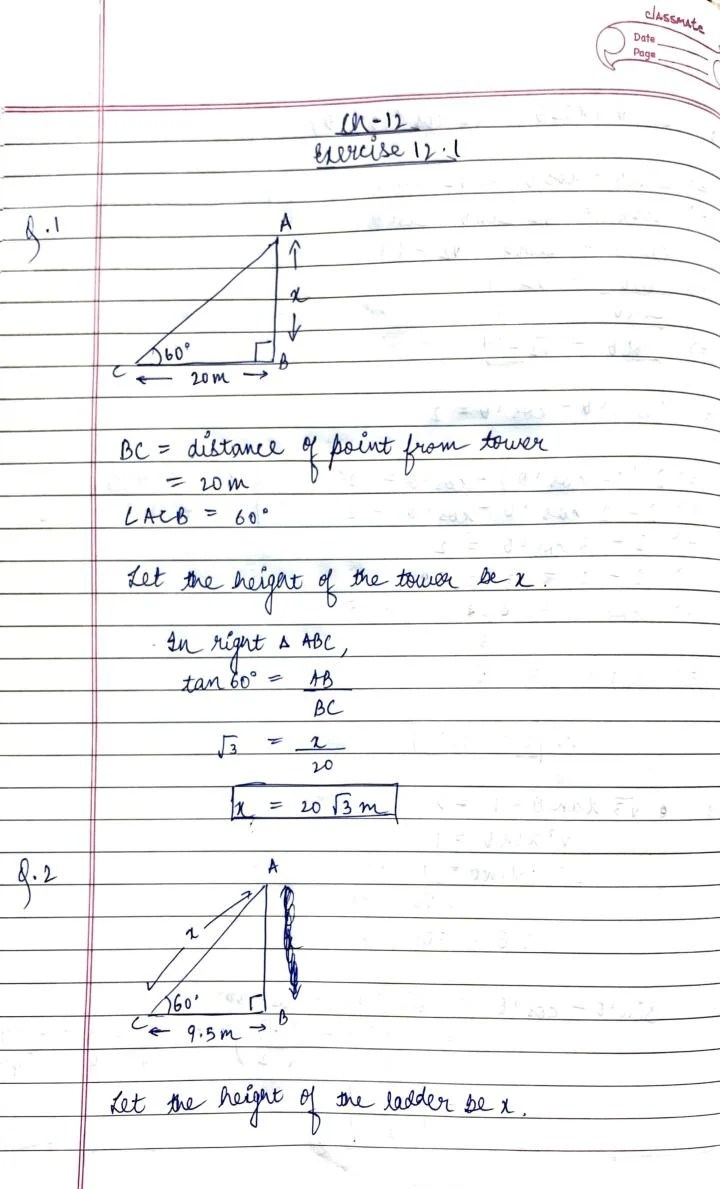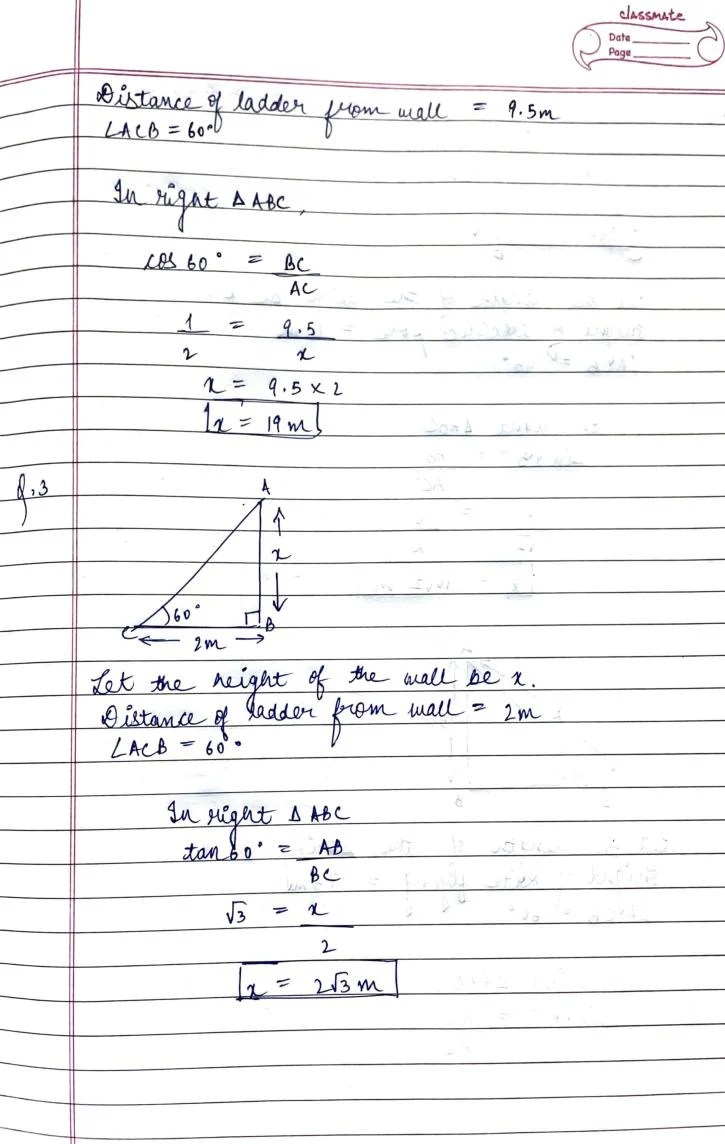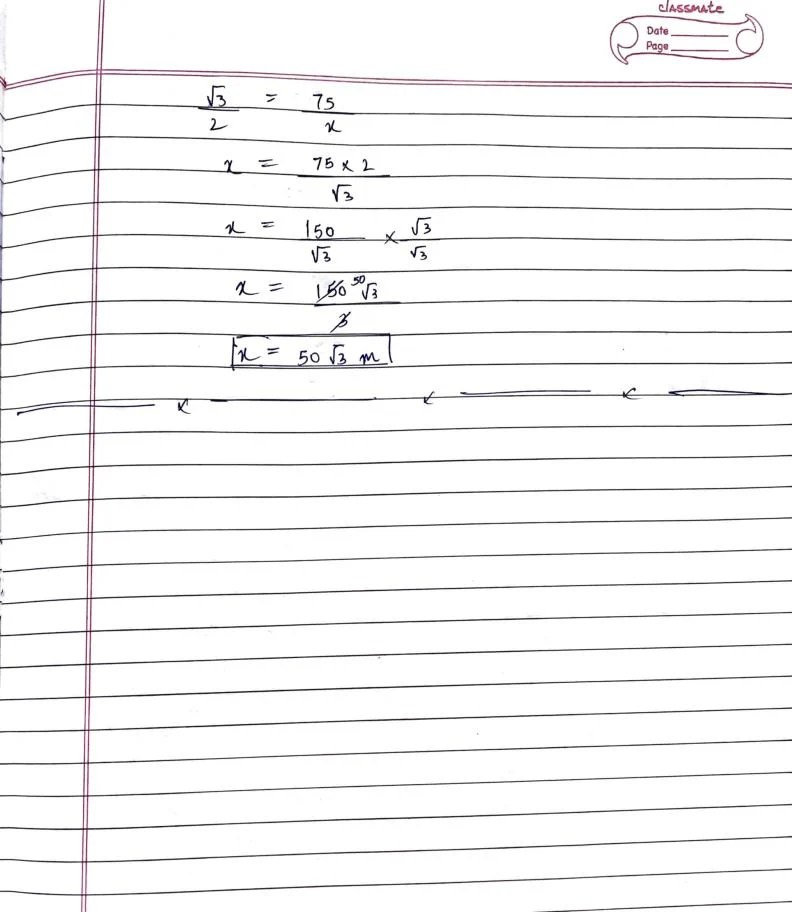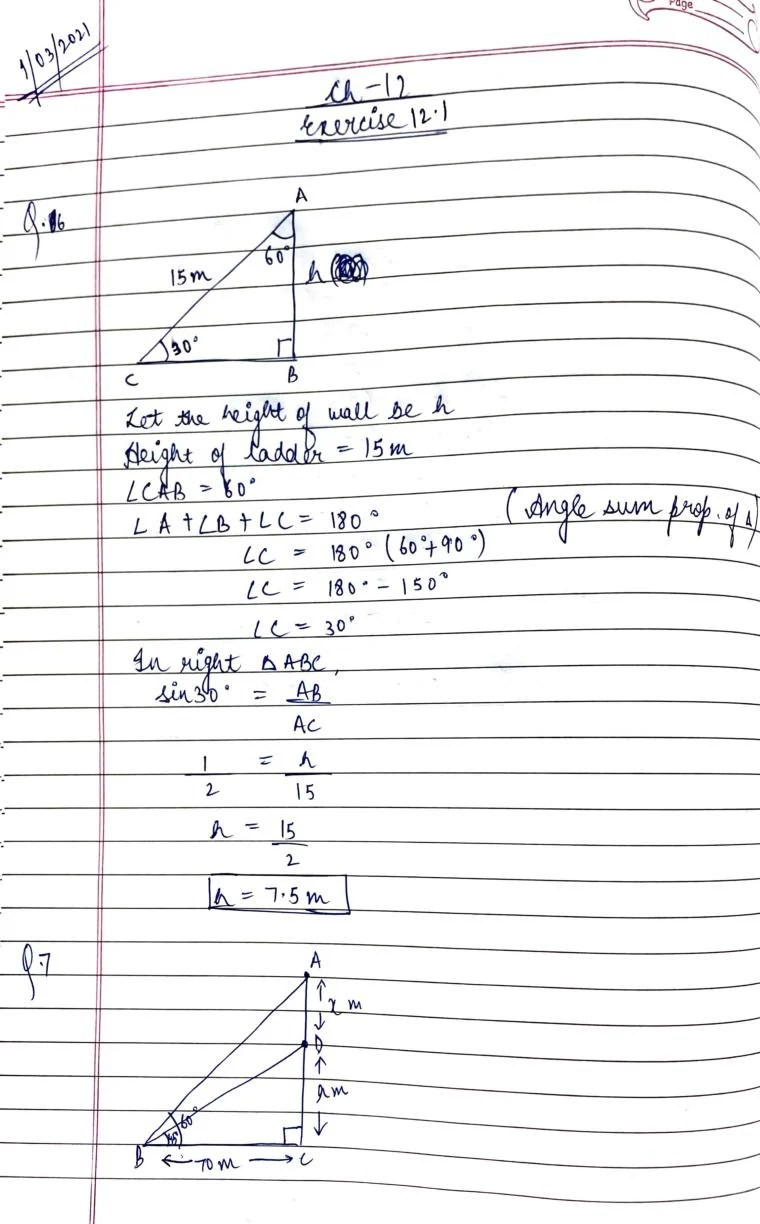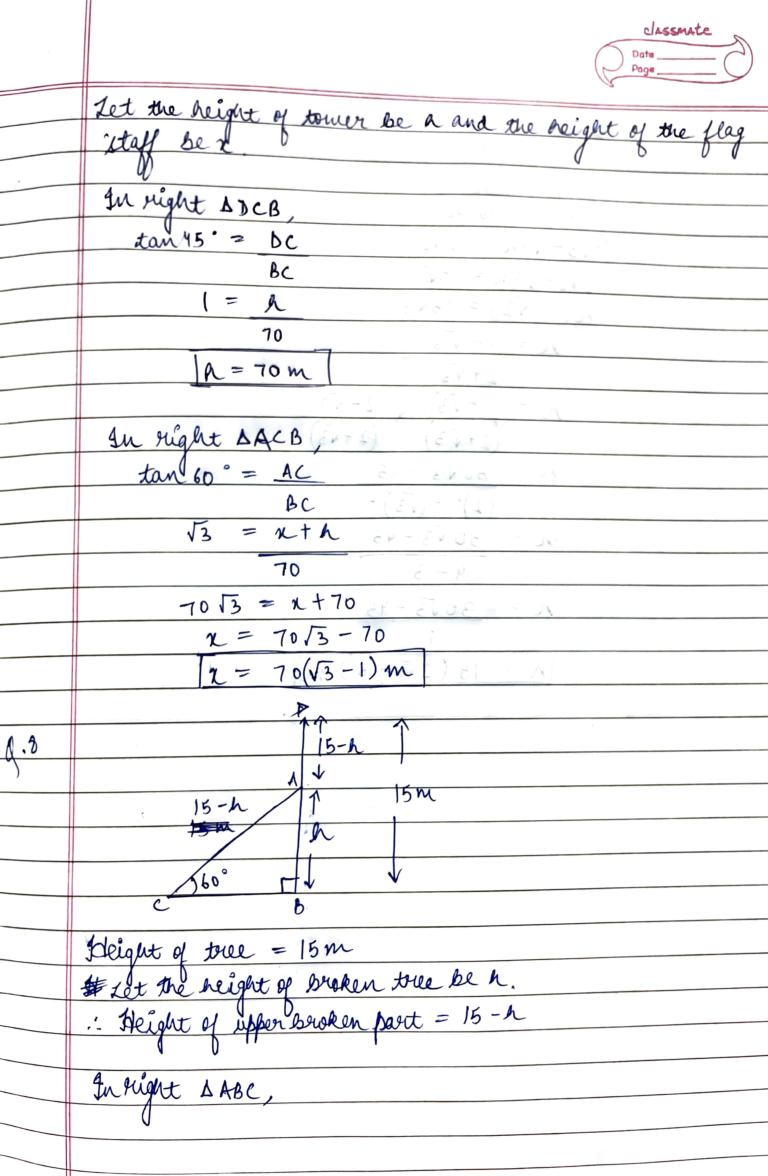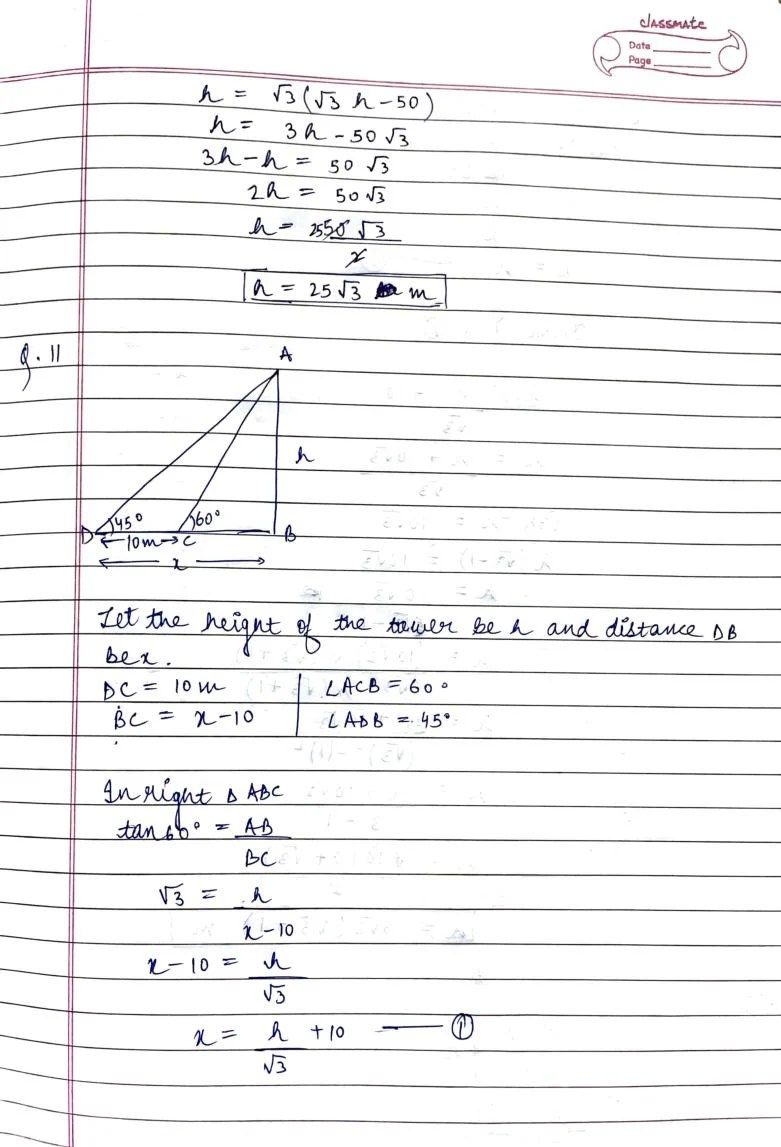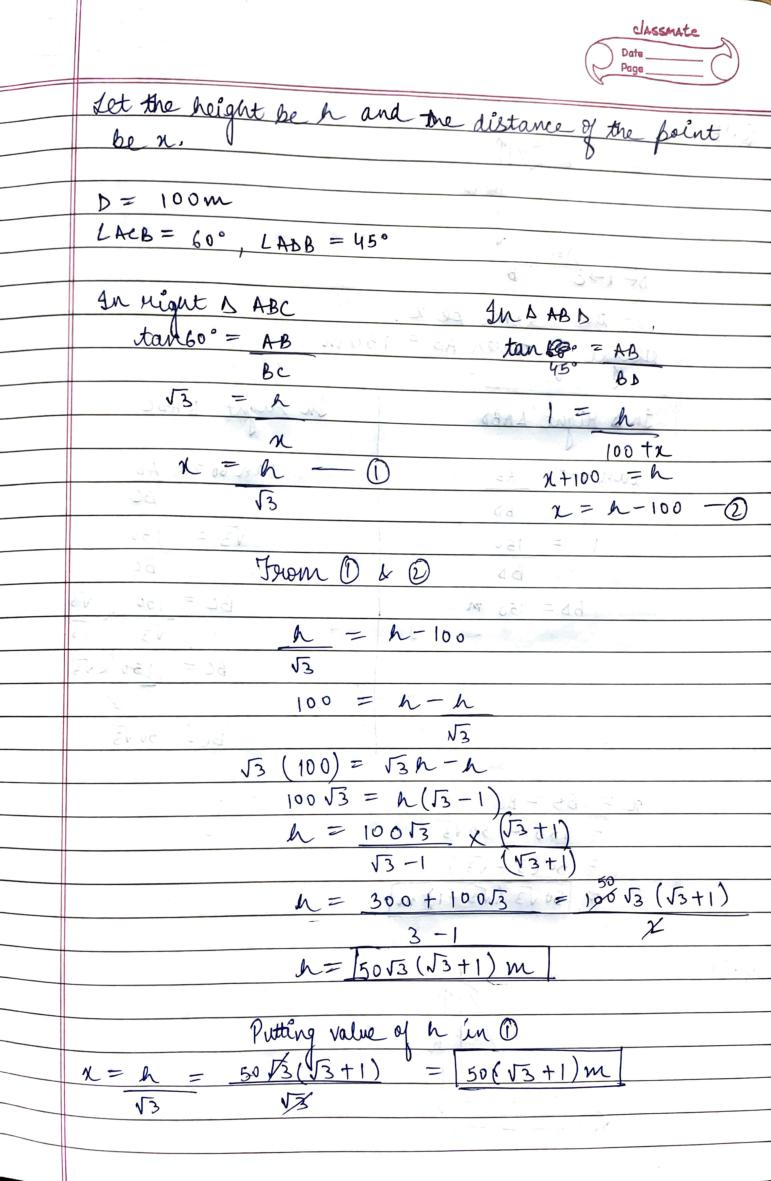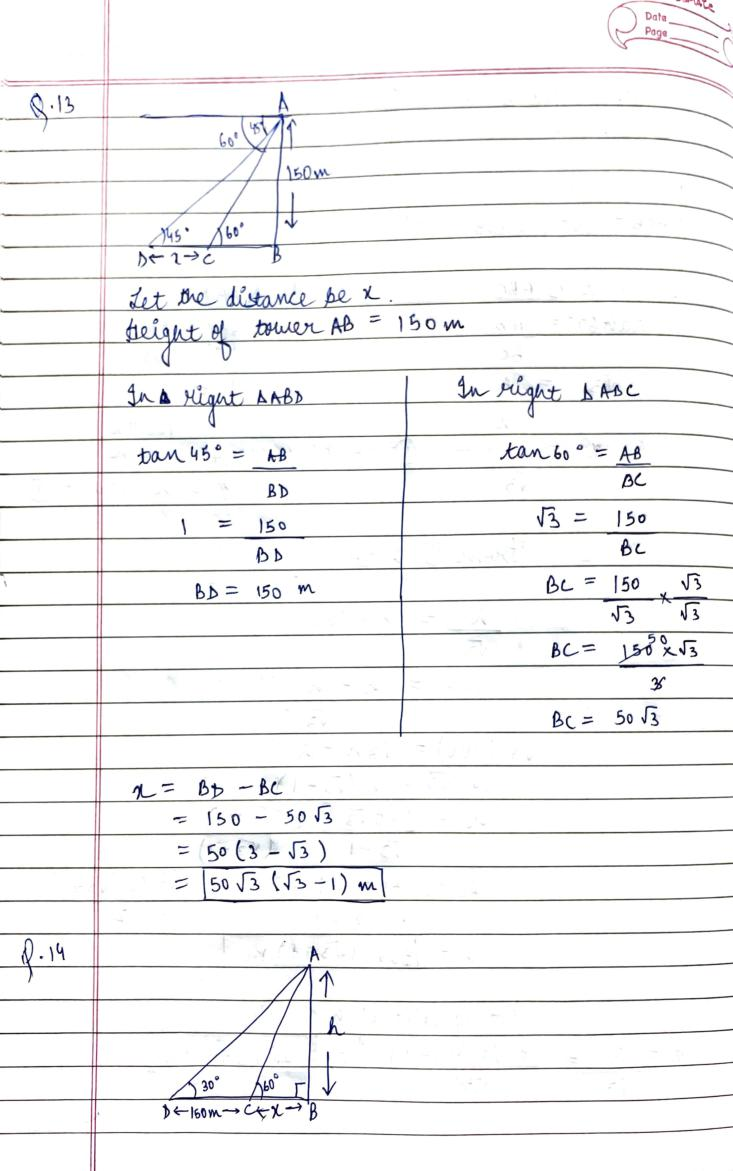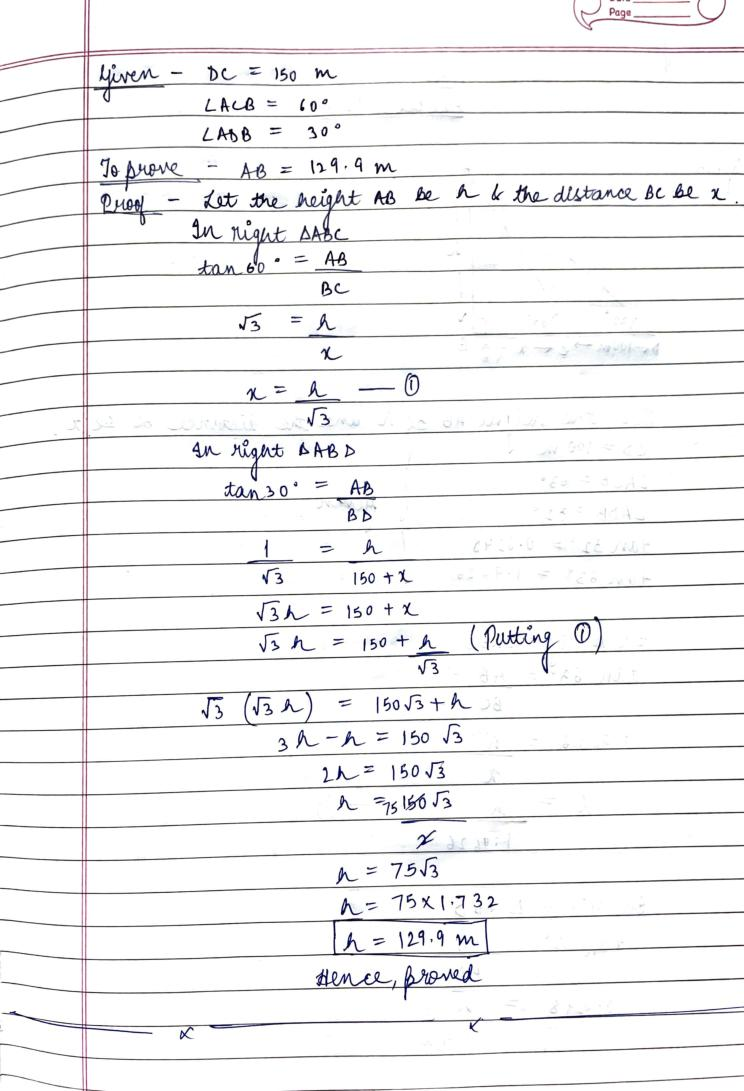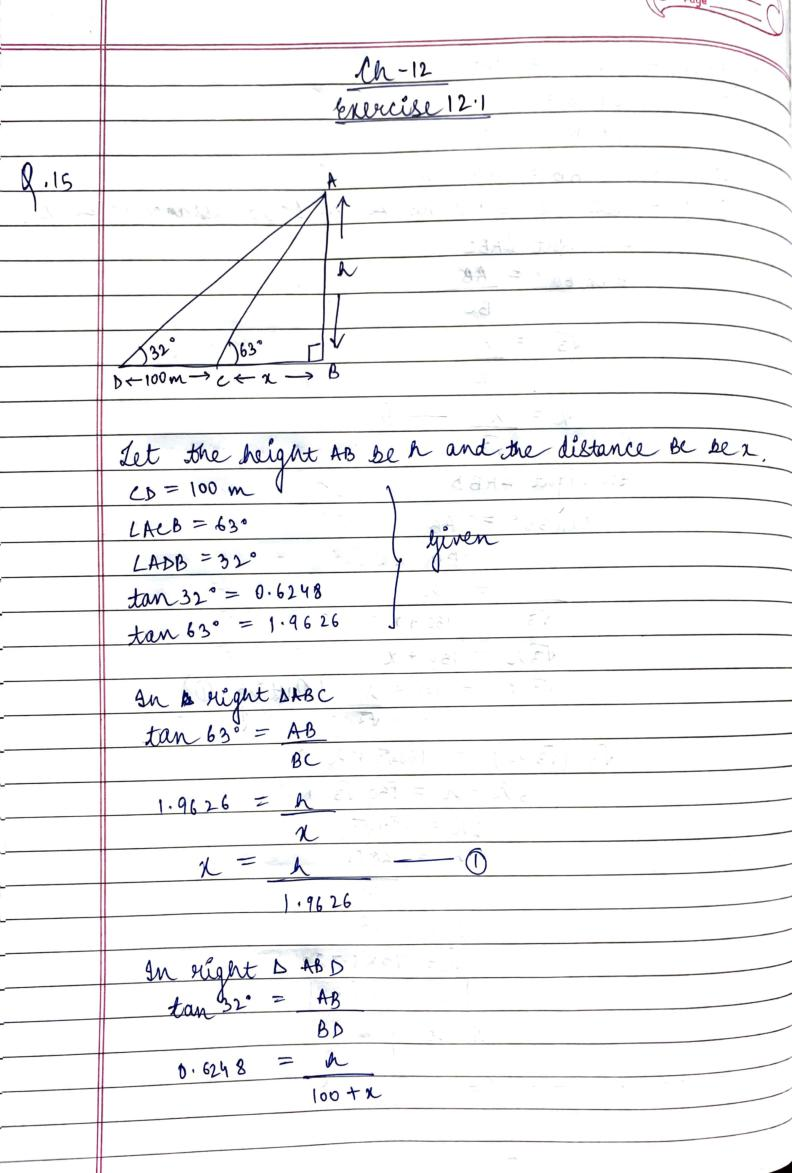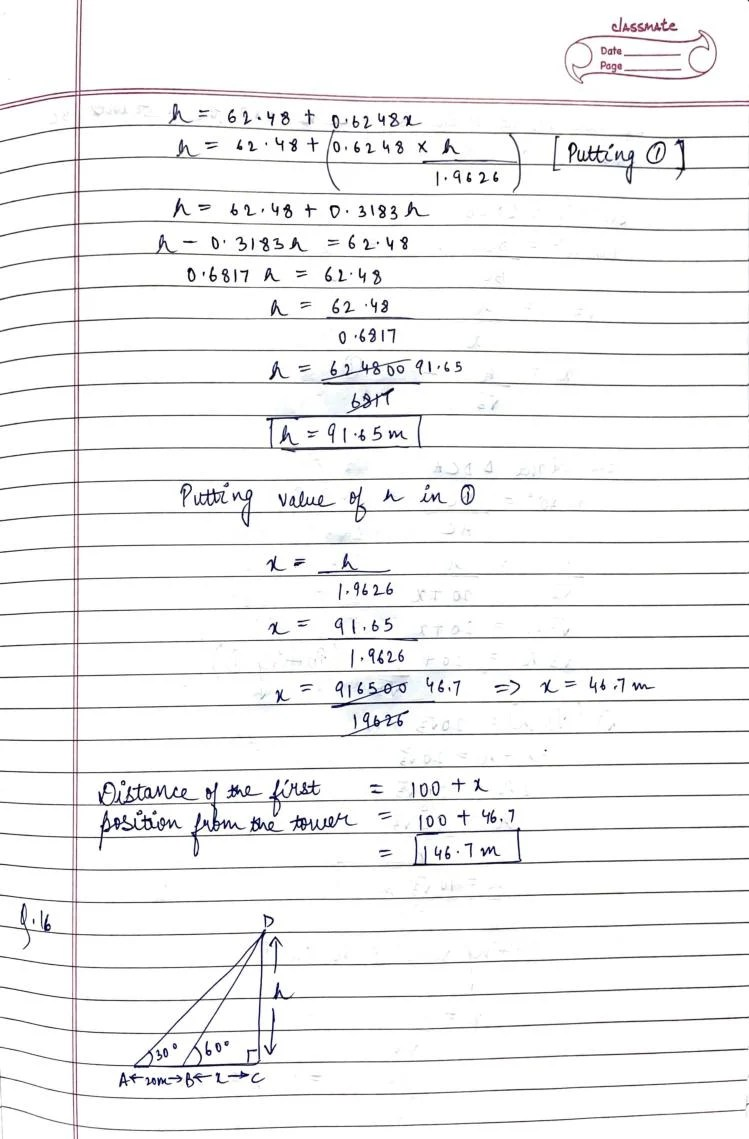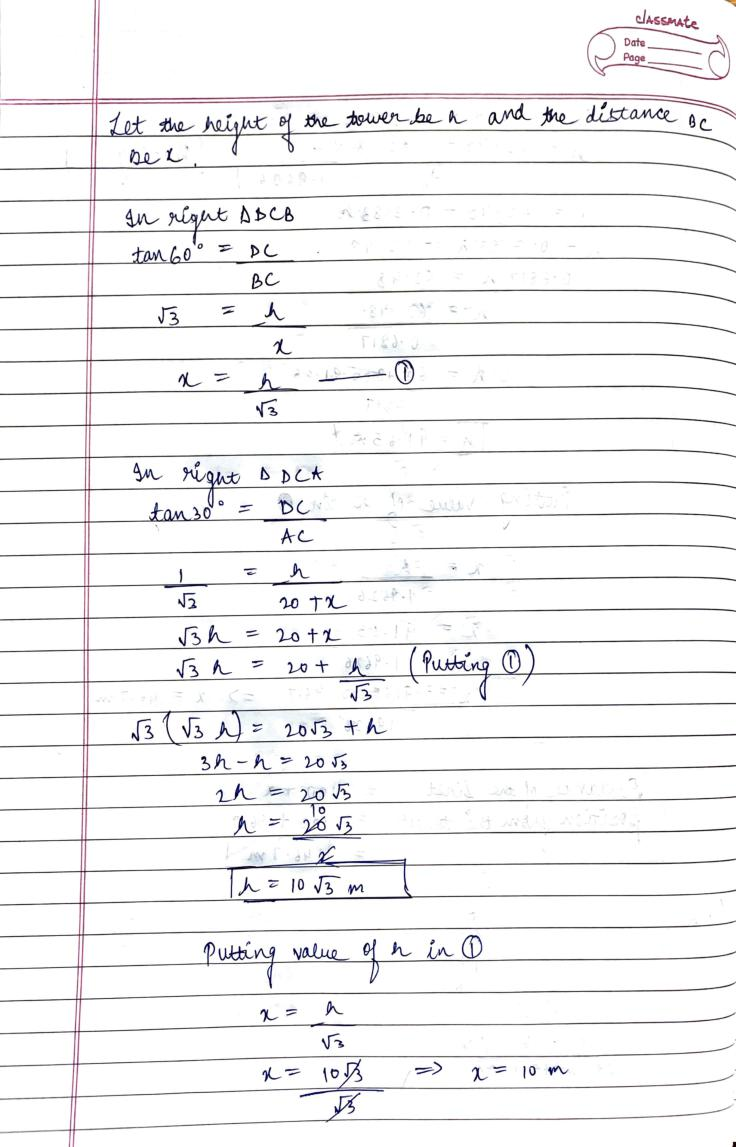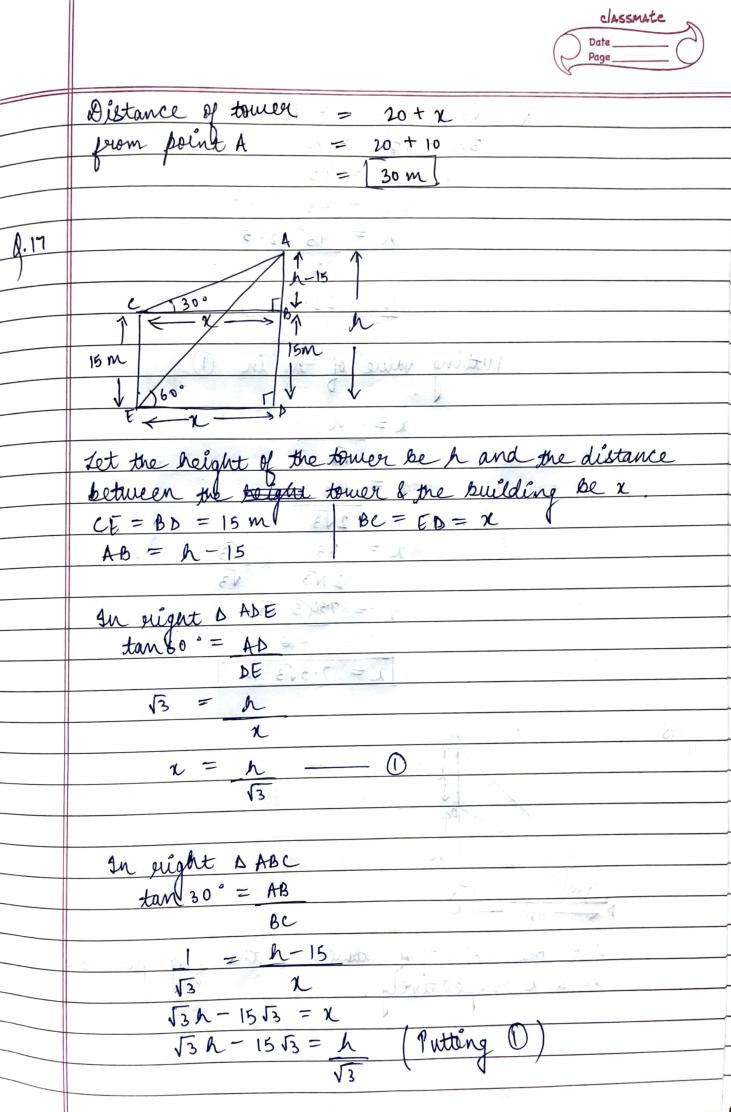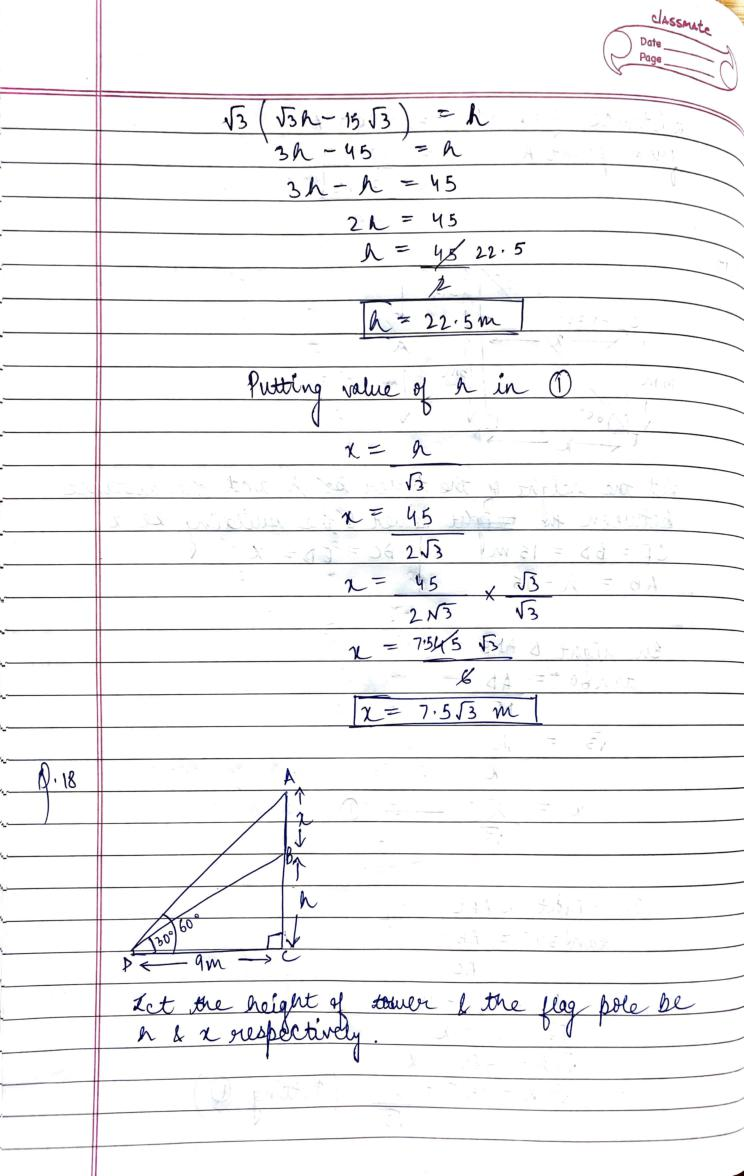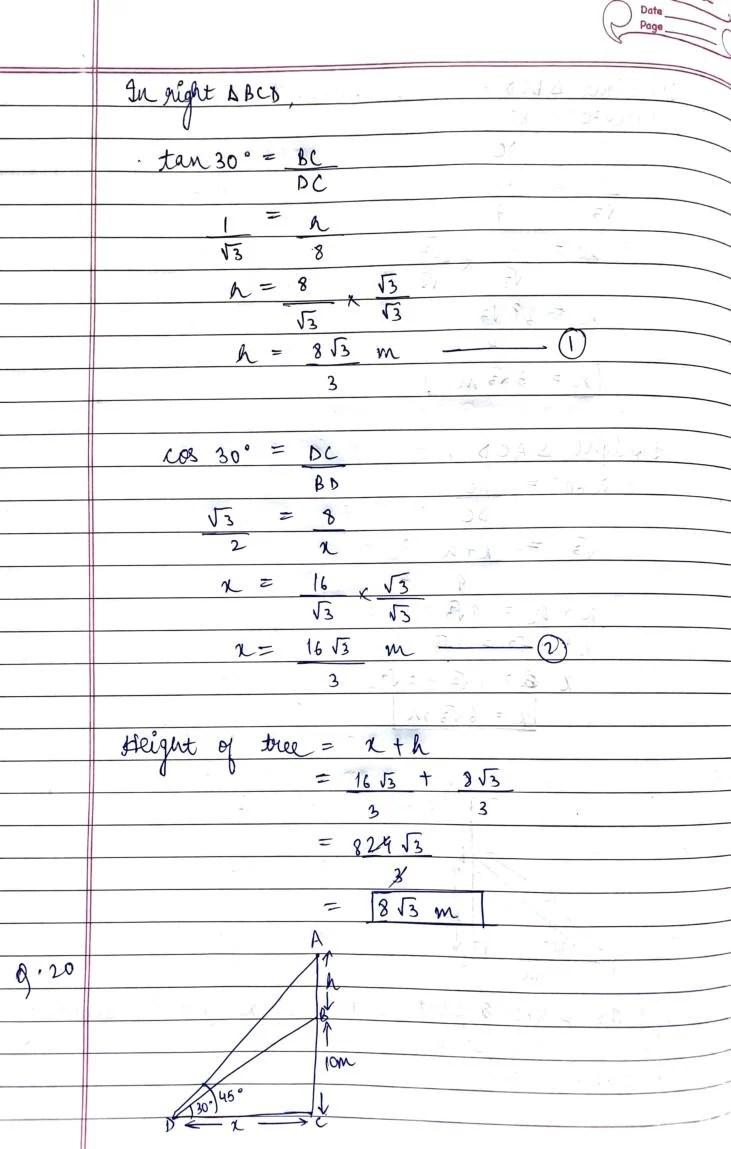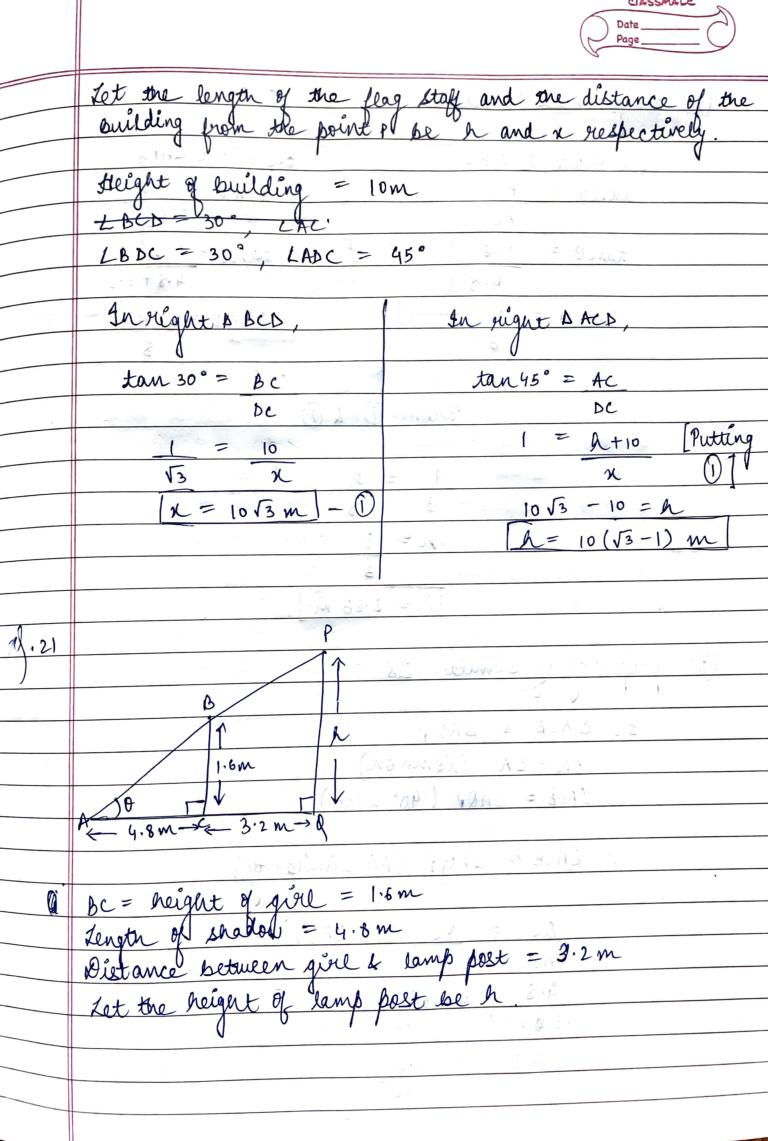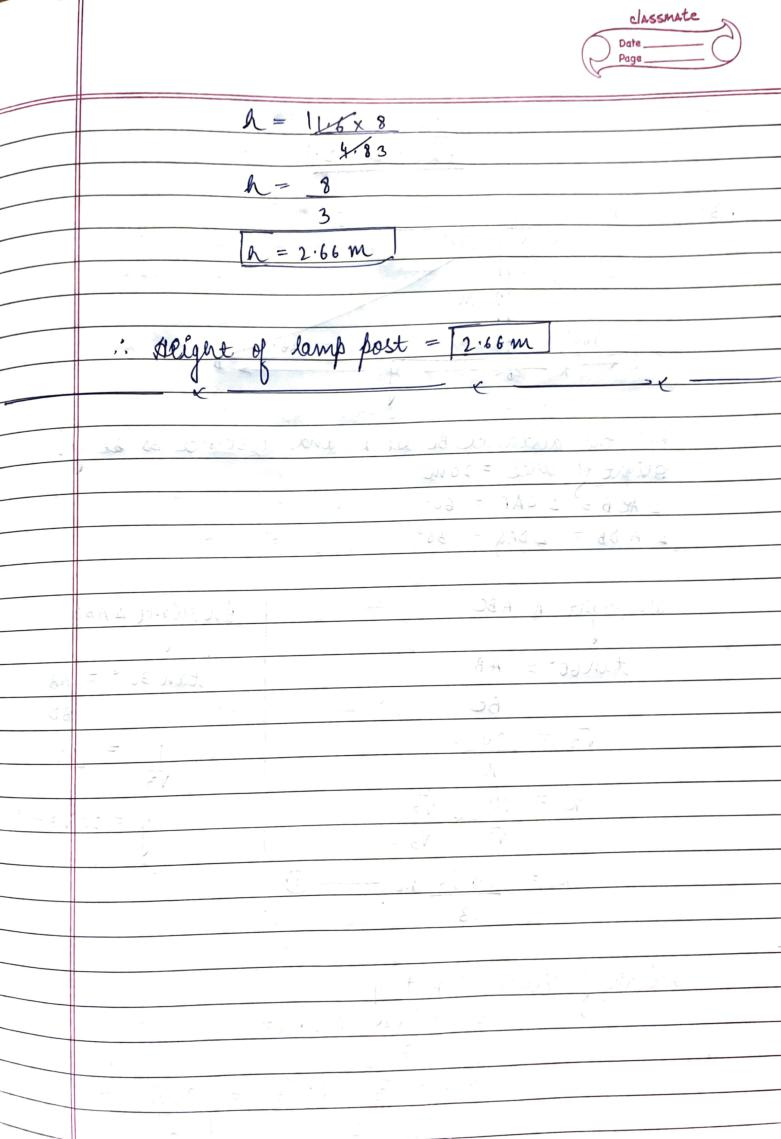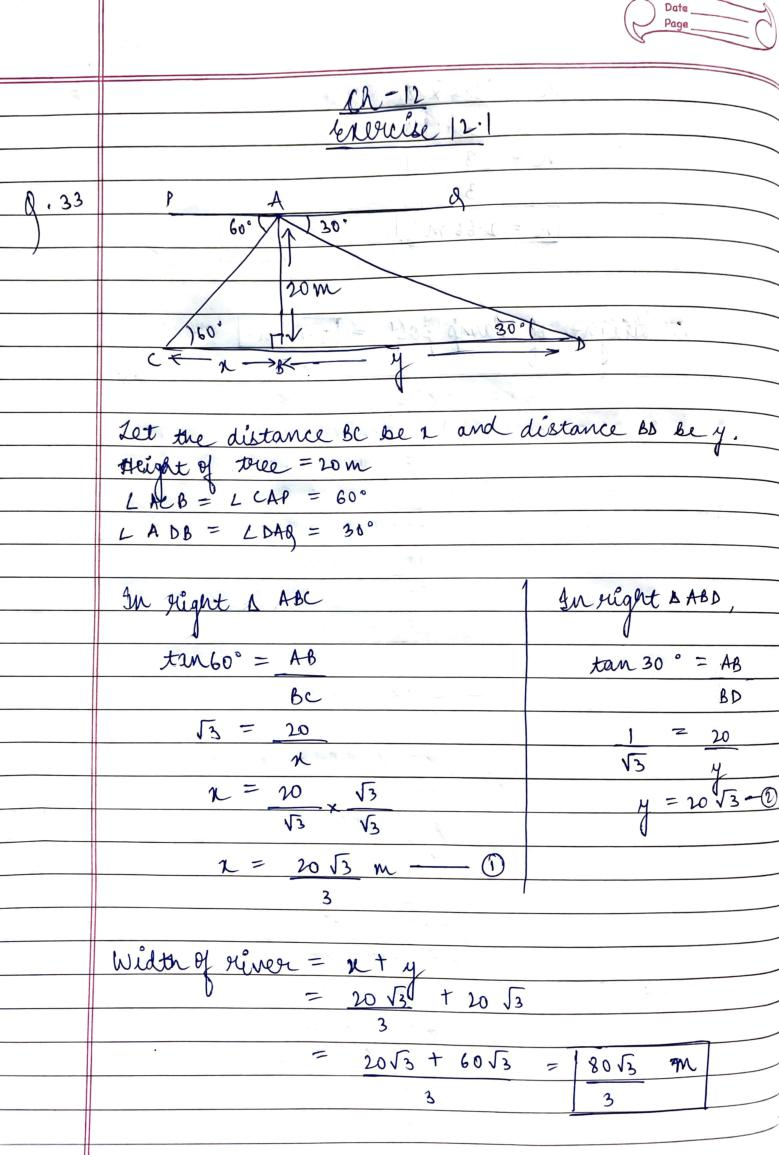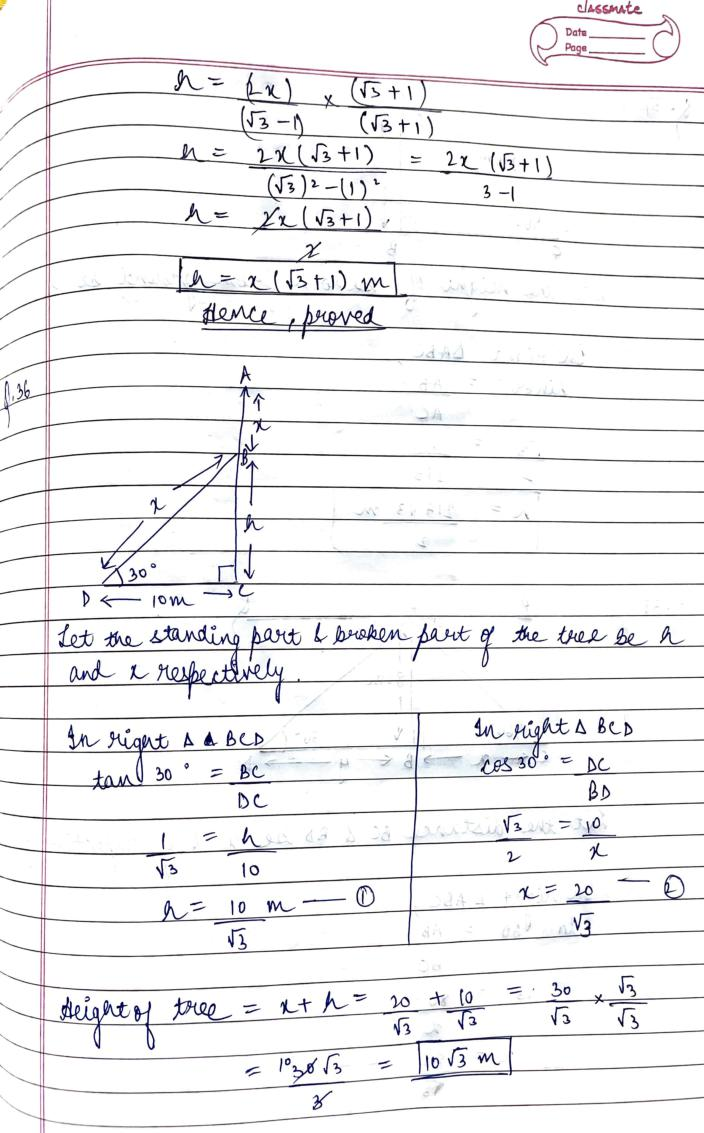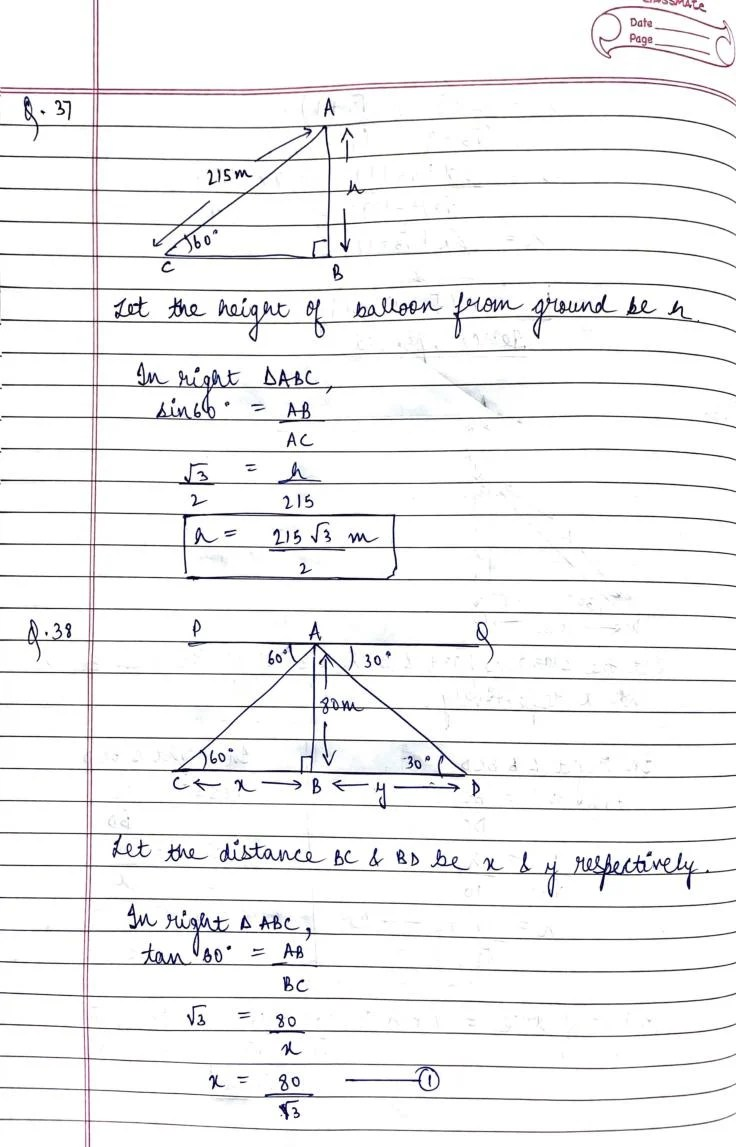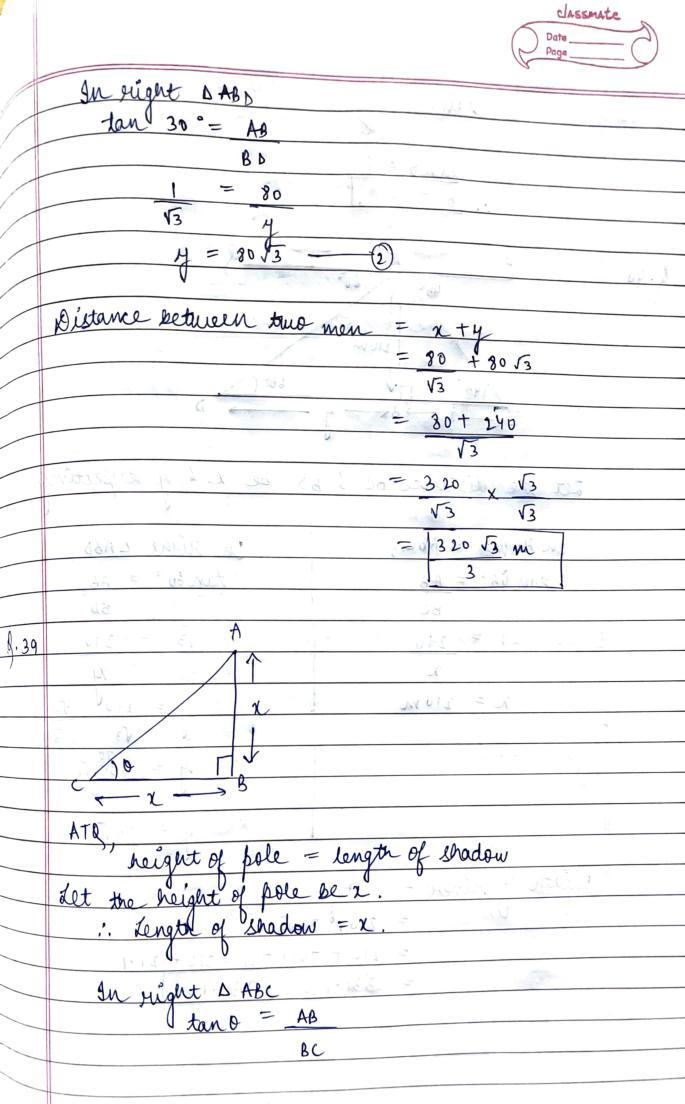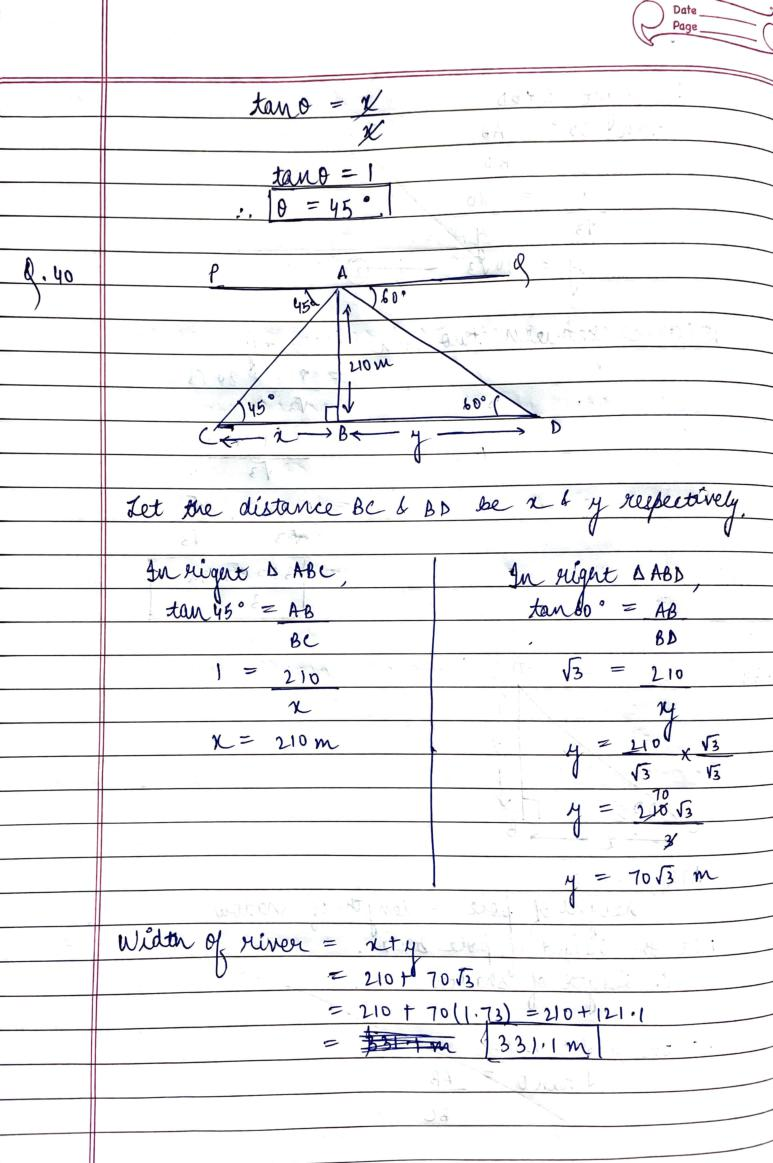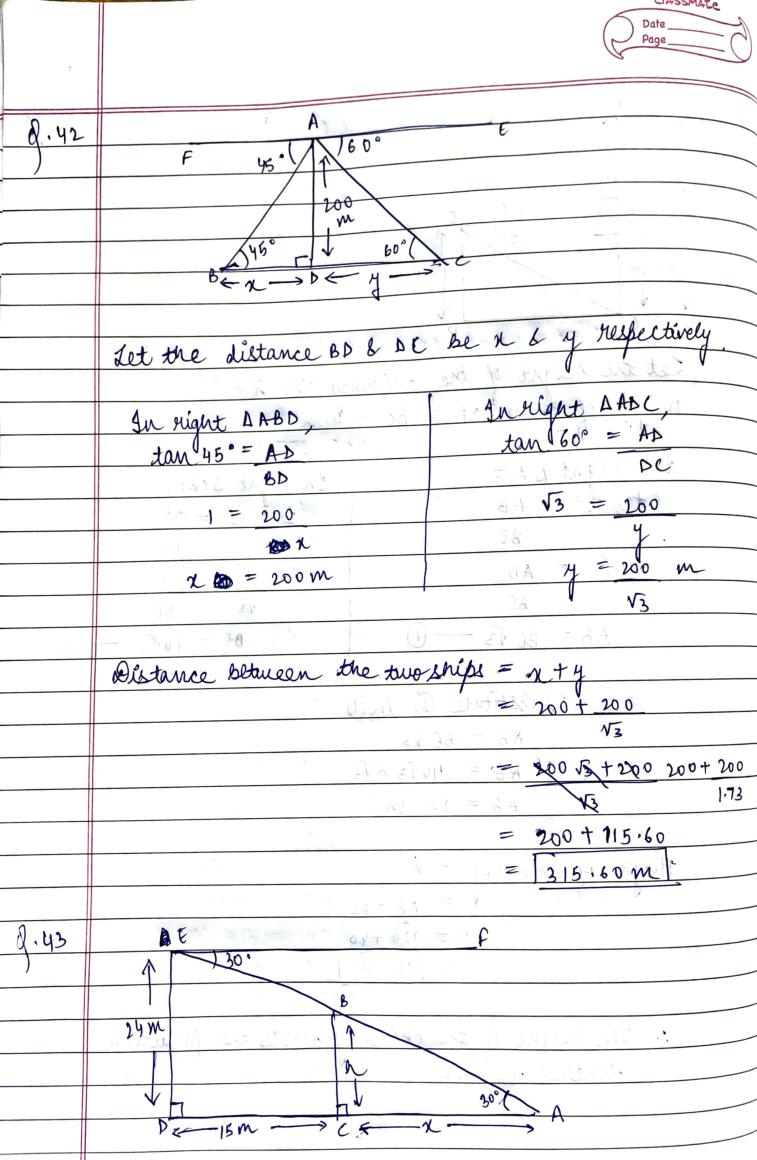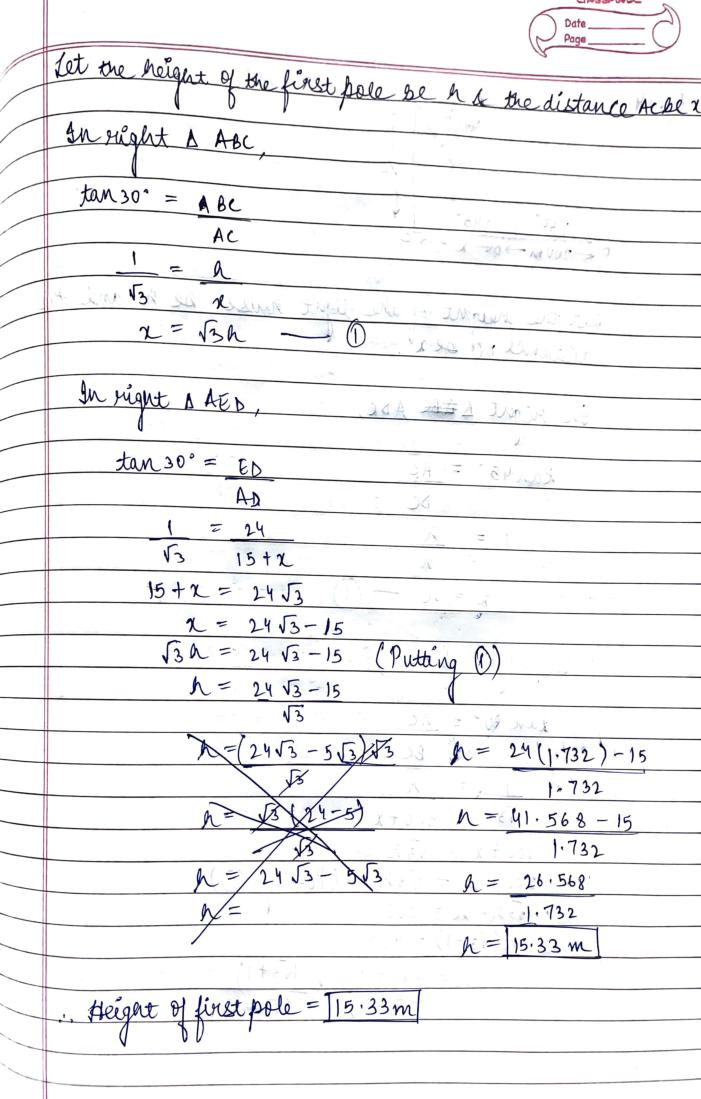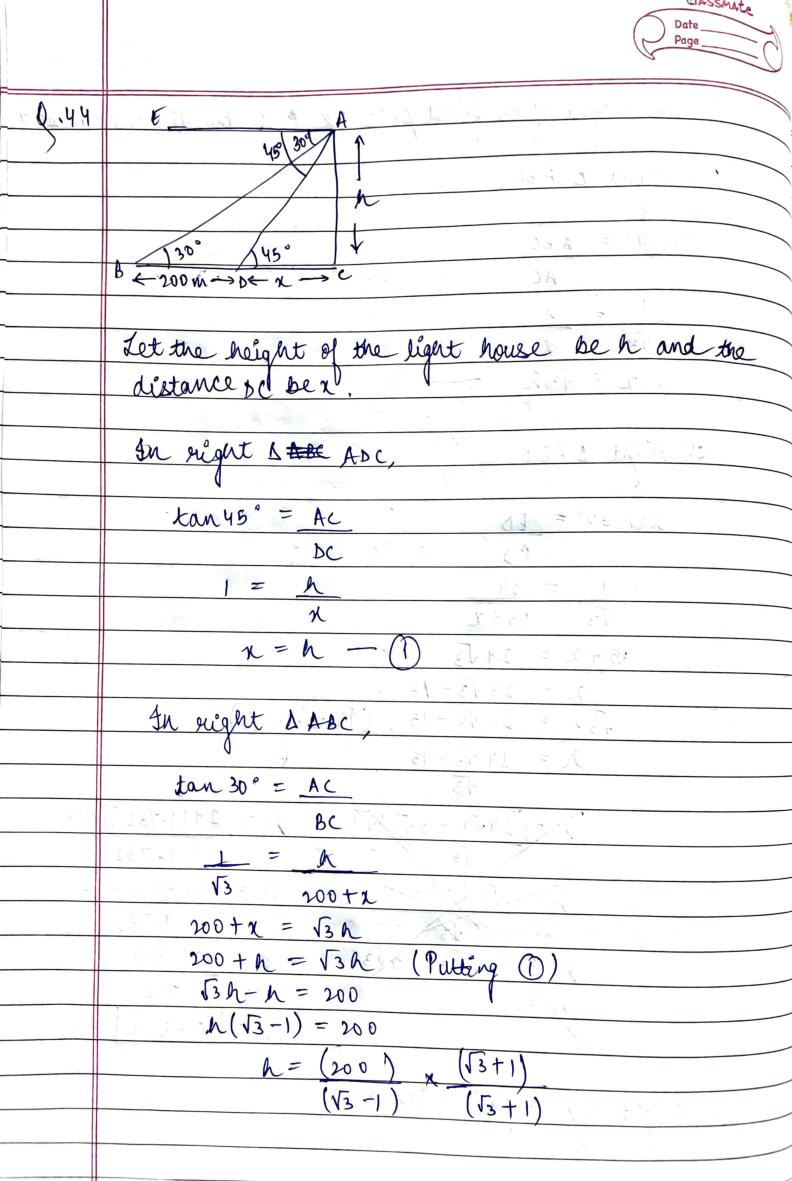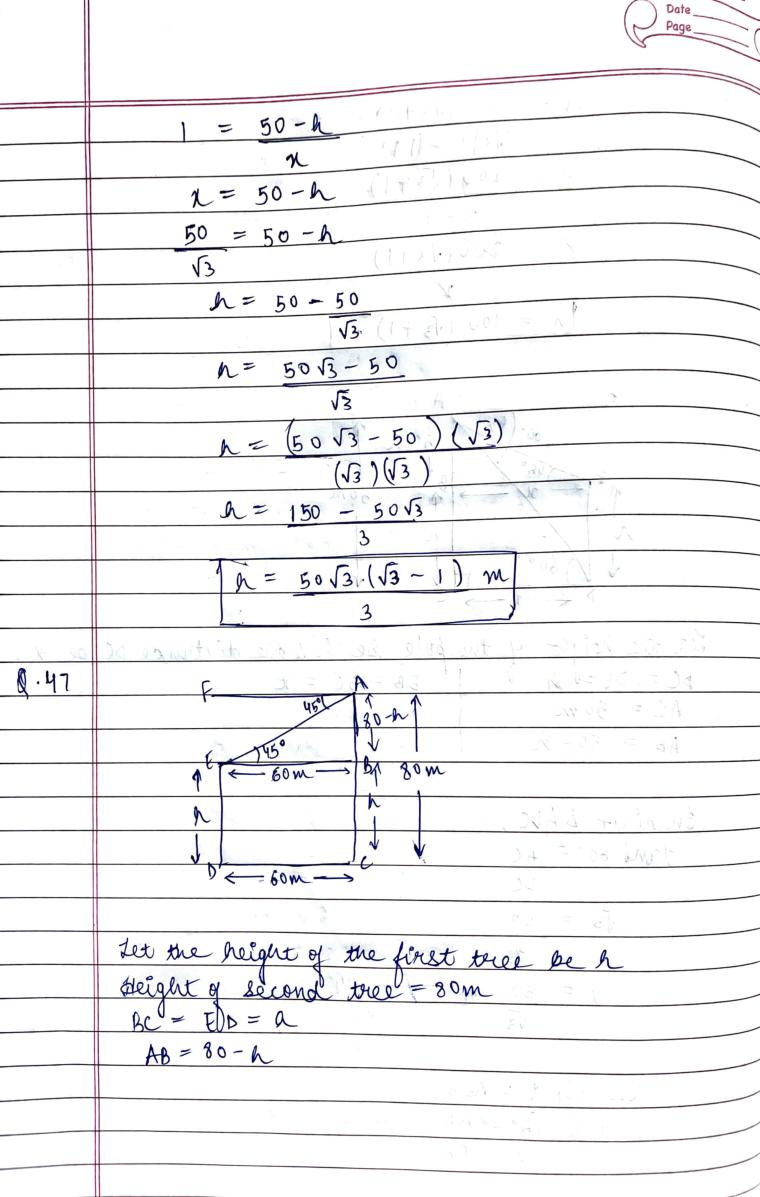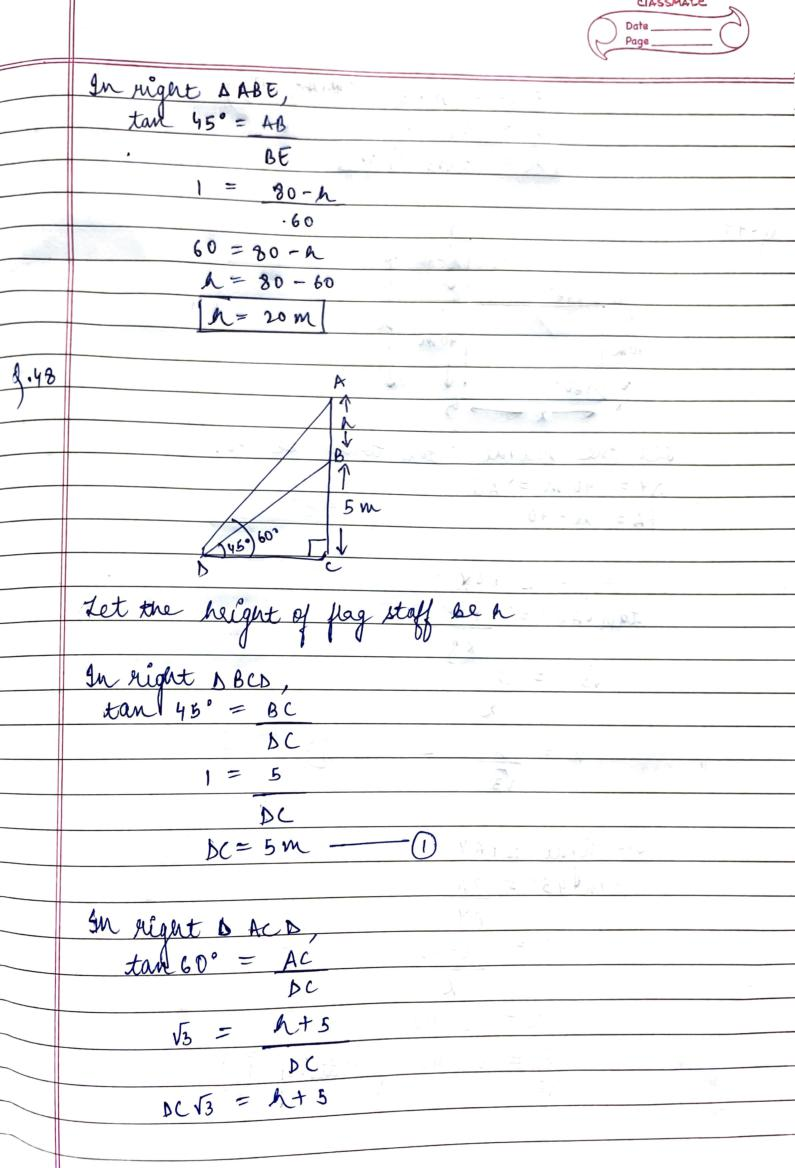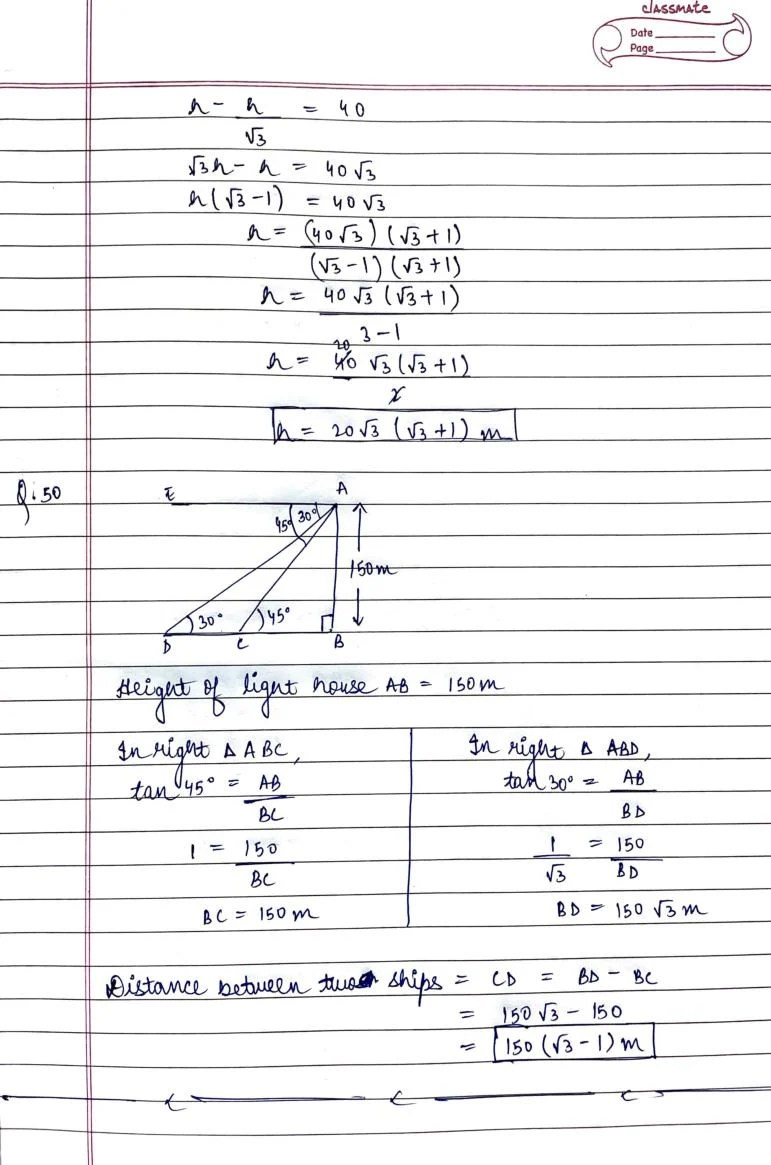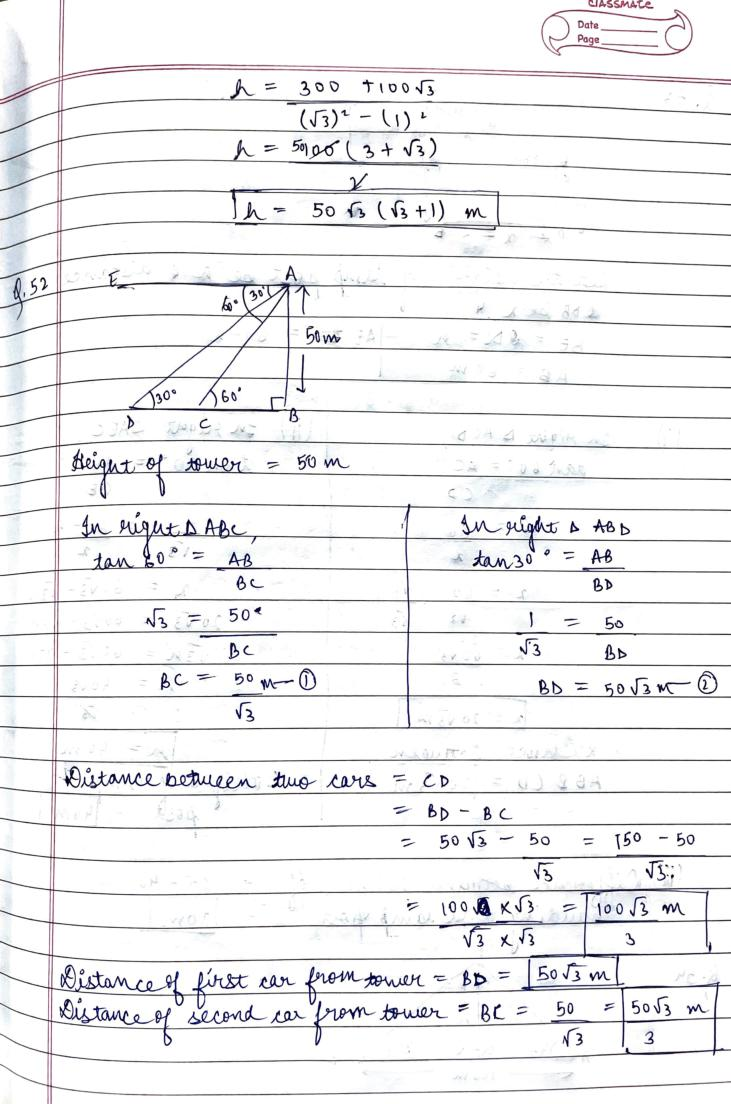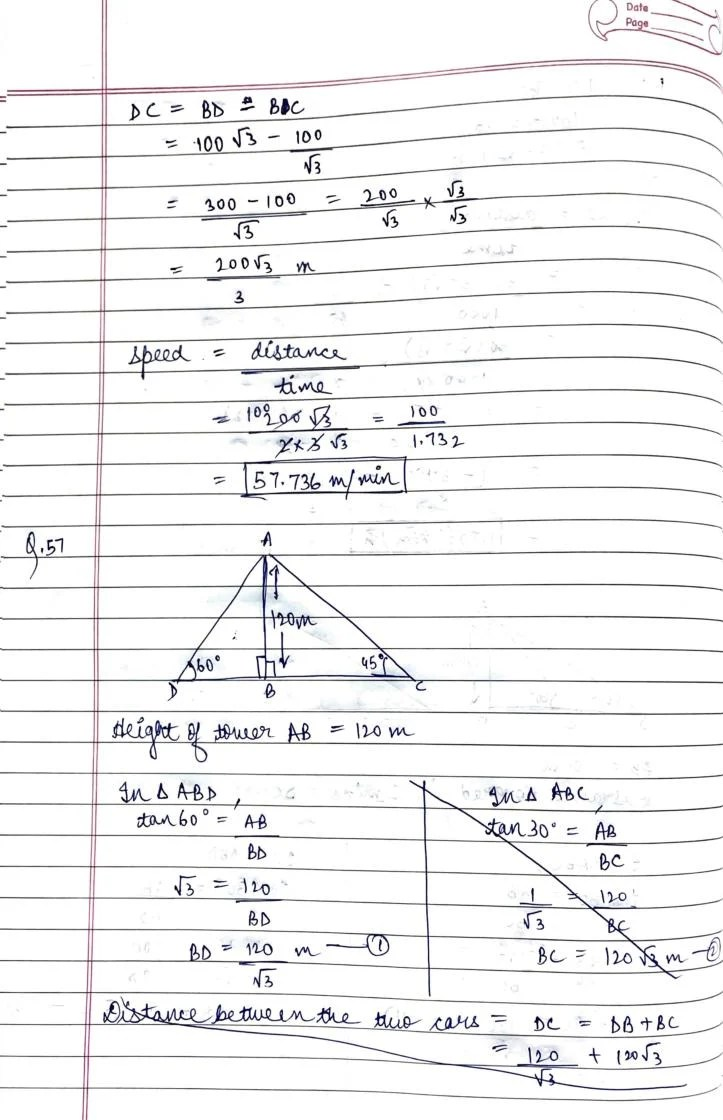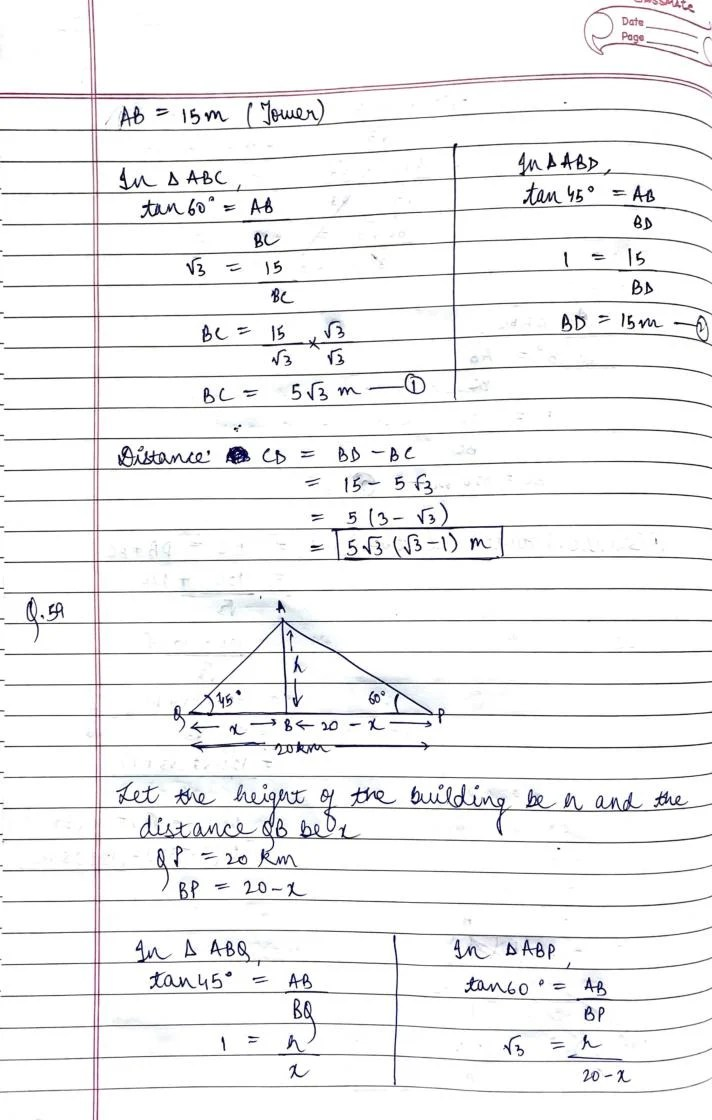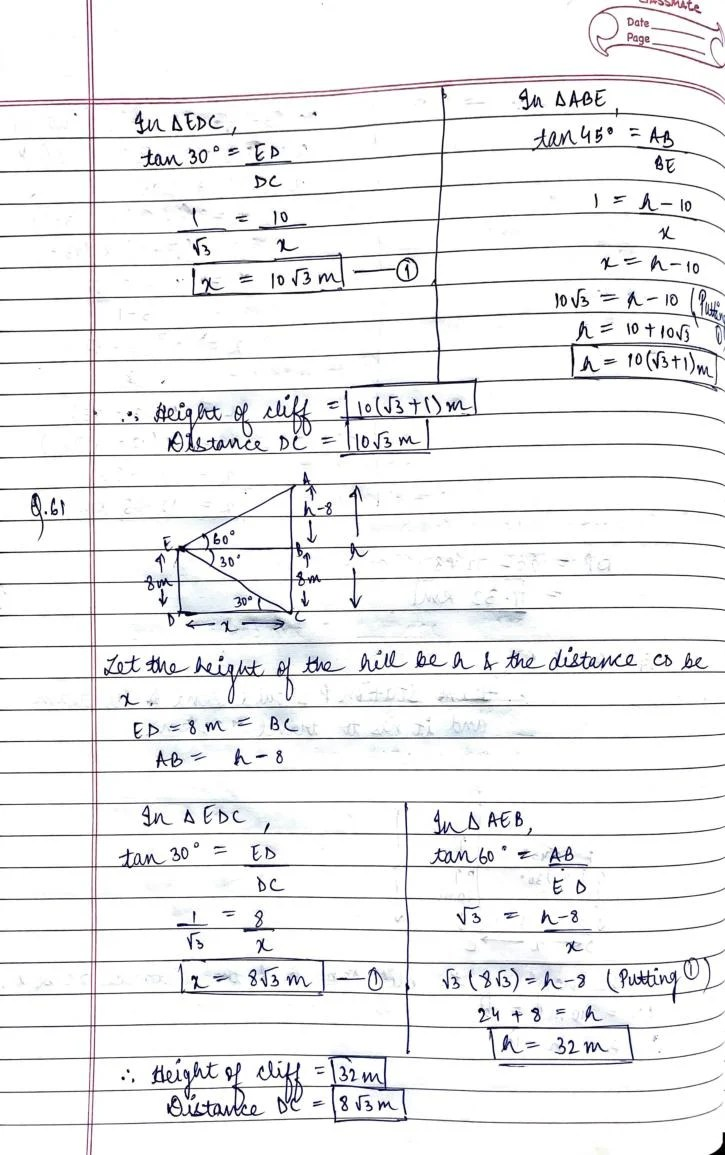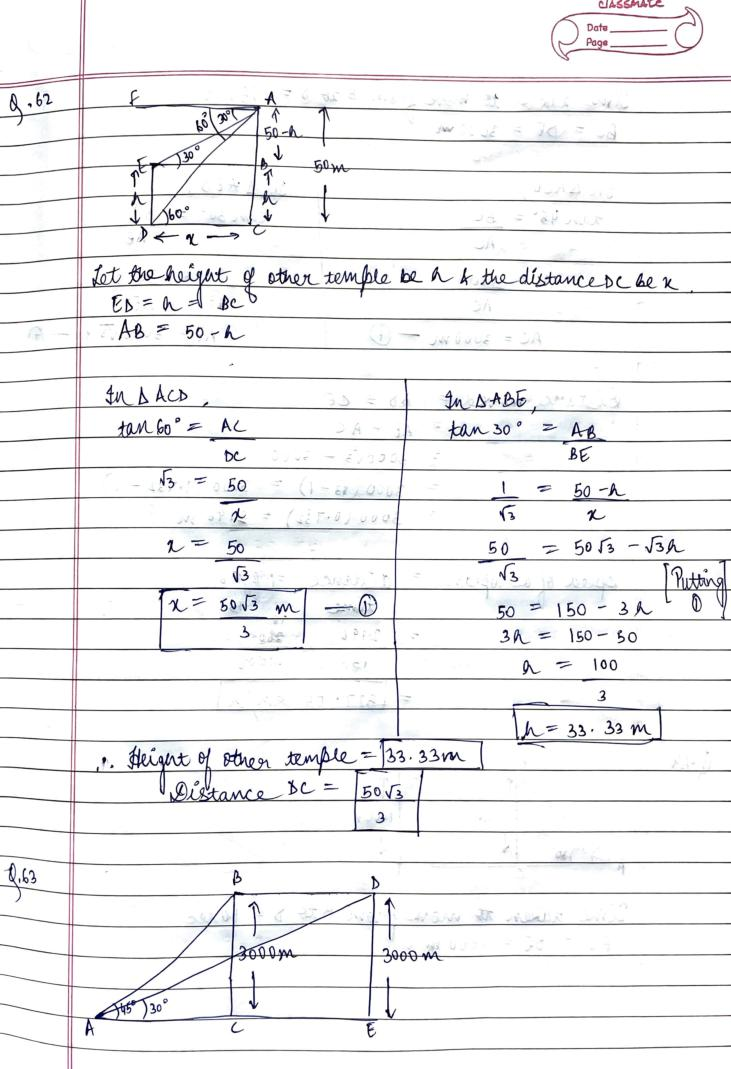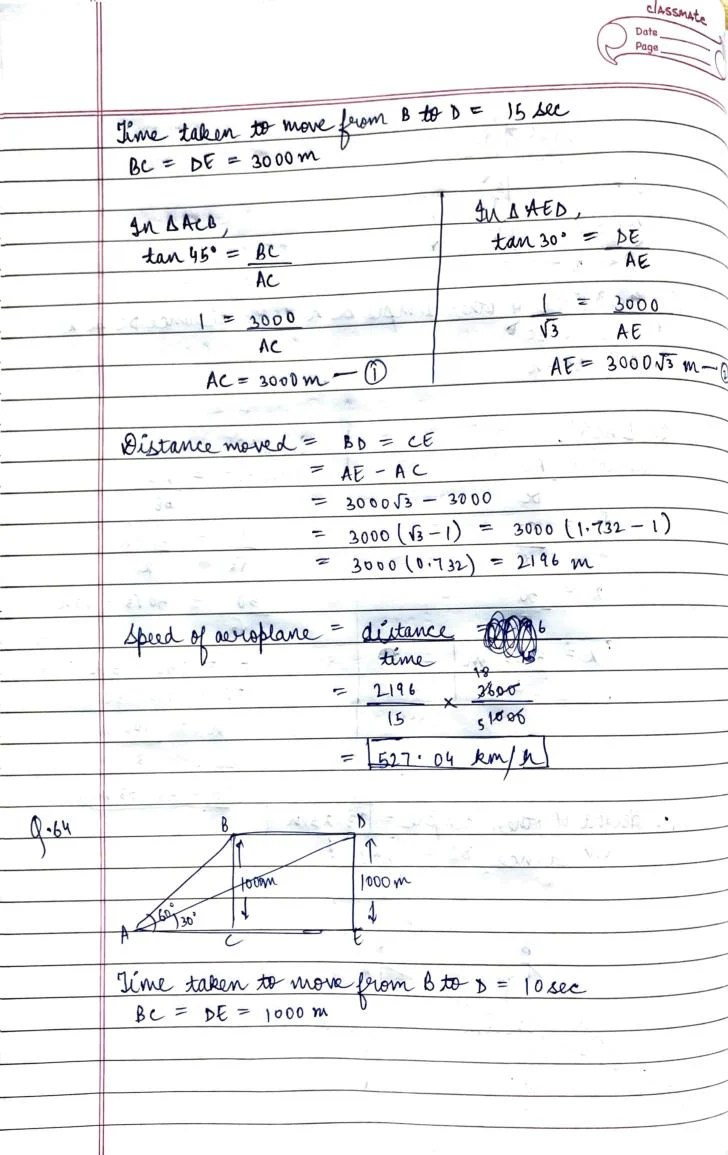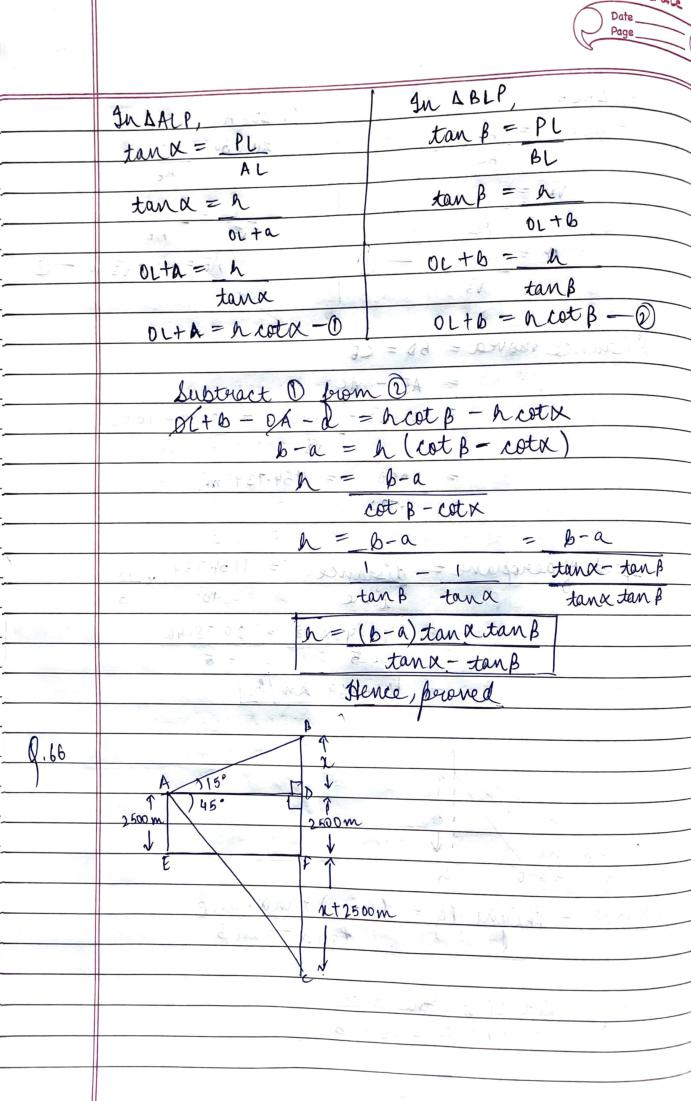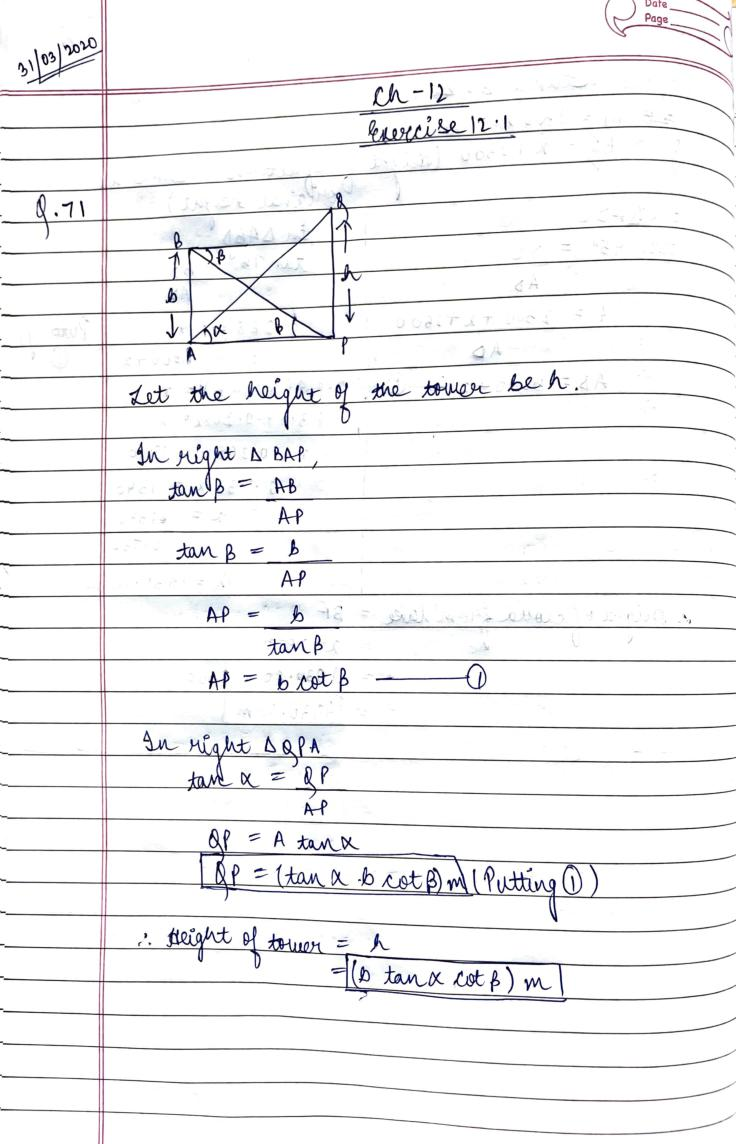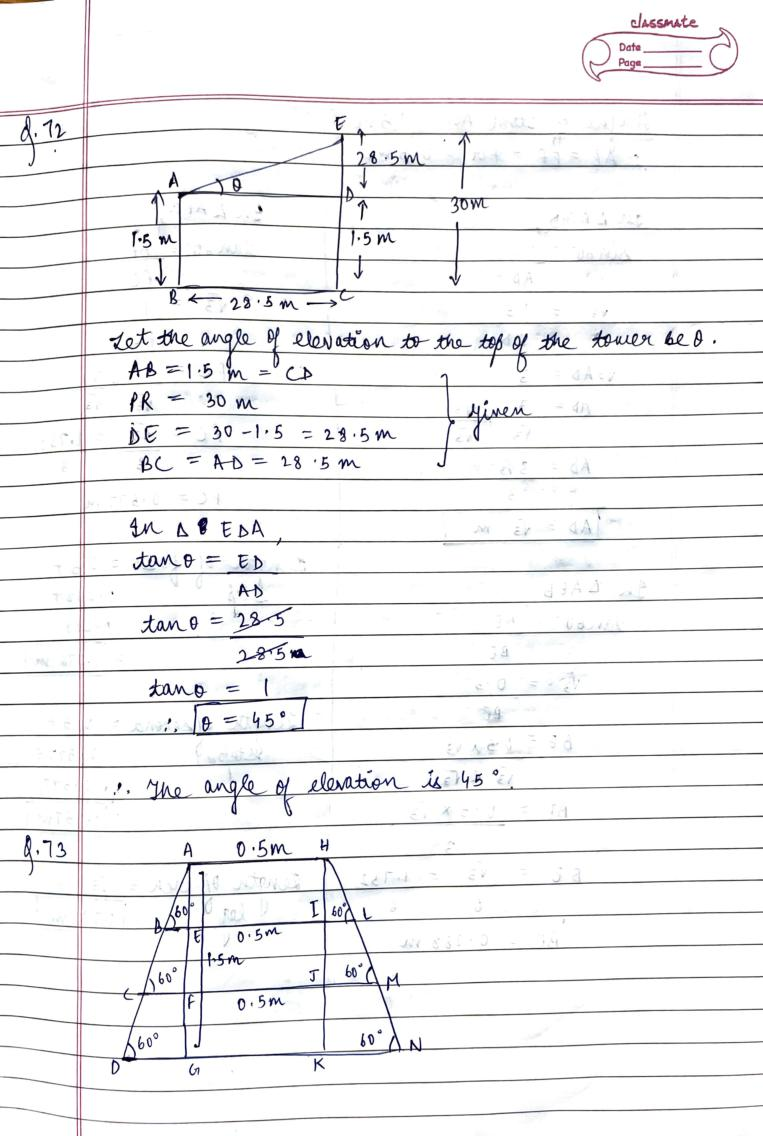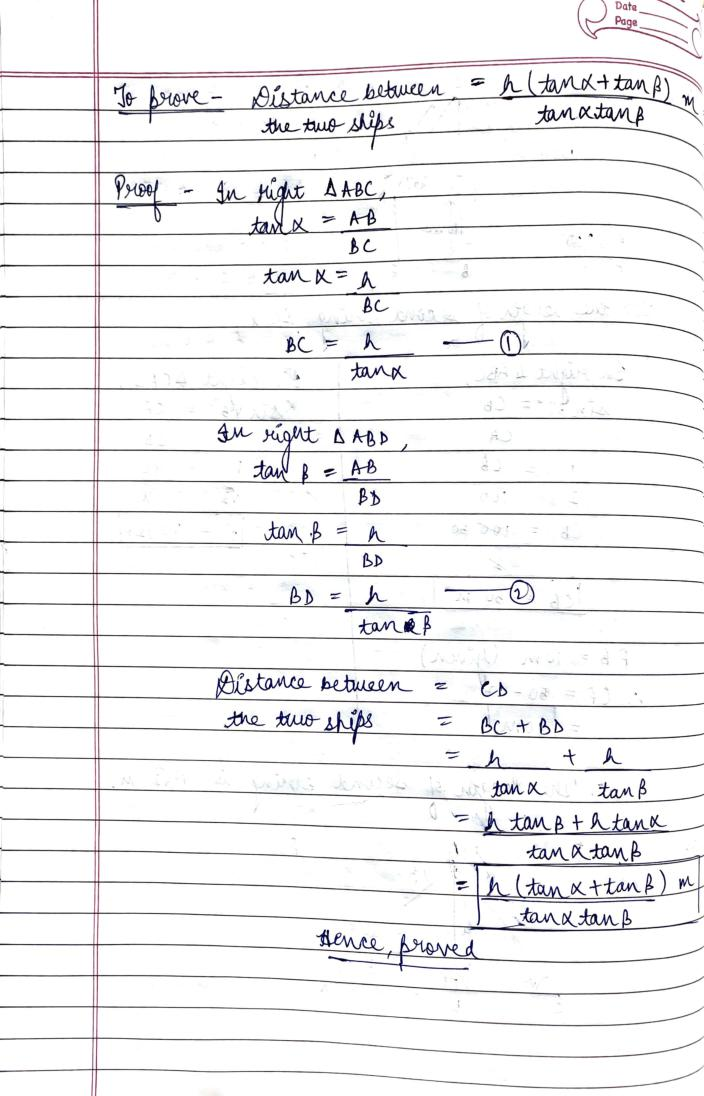RD Sharma Solutions for Class 10 Maths PDF download for Chapter 12 Some Applications of Trigonometry Exercise 12.1 is available here for free.

 « Previous Next »

### RD Sharma Solutions Class 10 Chapter 12 Exercise Wise

You can also see RD Sharma Solutions of all exercises of Chapter 12 – Some Applications of Trigonometry from here.

### RD Sharma Solutions Class 10 Chapter Wise

If you want RD Sharma solutions of any topic other than Chapter 12 Some Applications of Trigonometry, check it from here. Mathematics by RD Sharma has all chapters that are in your Class 10 syllabus.

Click to rate this post!
[Total: 1 Average: 5]

### 1 thought on “RD Sharma Solutions Class 10 Chapter 12 Some Applications of Trigonometry Exercise 12.1”

1.Thanks